## Seven Most Popular SVM Kernels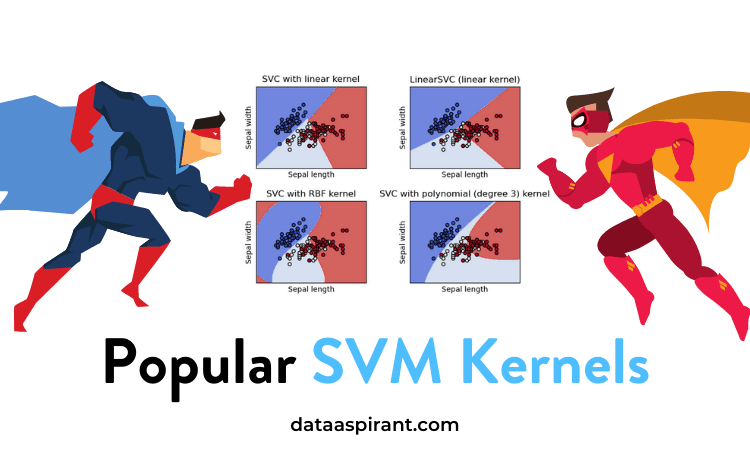While explaining the support vector machine, SVM algorithm, we said we have various svm kernel functions that help changing the data dimensions.

So In this article, we are going to dive deep into svm algorithm and SVM’s kernel functions.

Let me give you a quick introduction of svm and its kernels.

We already know that SVM is a supervised machine learning algorithm used to deal with both classification and regression problems. Compared to the other classification and regression algorithms, the svm approach is completely different.

One key reason for this is svm kernel functions.

Learn about the most popular SVM kernels along with the implementation in python #svm #svmkernels #classification #regression #machinelearning #datascience #python

Kernel plays a vital role in classification and is used to analyze some patterns in the given dataset. They are very helpful in solving a no-linear problem by using a linear classifier

Later the svm algorithm uses kernel-trick for transforming the data points and creating an optimal decision boundary. Kernels help us to deal with high dimensional data in a very efficient manner.

We have various svm kernel functions to convert the non-linear data to linear. In this article, we listed 7 such popular svm kernel functions.

Before we drive further, let’s have a look at the topics you are going to learn in this article.

Let’s start the article with SVM. If you are interested in the sum algorithm implementation in python and R programming language, please refer to below two articles.

## What Is the Support Vector Machine (SVM)?SVM is a famous supervised machine learning algorithm used for classification as well as regression algorithms. However,  mostly it is preferred for classification algorithms. It basically separates different target classes in a hyperplane in n-dimensional or multidimensional space.

The main motive of the SVM is to create the best decision boundary that can separate two or more classes(with maximum margin) so that we can correctly put new data points in the correct class.

Wait!

Why is it known as SVM?

Because It chooses extreme vectors or support vectors to create the hyperplane, that’s why it is named so. In the below sections let’s understand in more detail.

## SVM Algorithm Explanation

To understand the SVM algorithm, we need to know about hyperplanes and support vectors.### SVM Hyperplane

There may be multiple lines/decision boundaries to segregate the classes in n-dimensional space. Still, we want to search out the simplest decision boundary that helps to classify the information points.

This best boundary is considered to be the hyperplane of SVM. The dimensions of the hyperplane rely on the features present within the dataset. These features suggest if there are 2 target labels in the dataset.

Then the hyperplane is going to be a line. And if there are 3 target labels, then the hyperplane is going to be a 2-dimension plane. We always create a hyperplane that provides maximum margin. This margin simply means there should be a maximum distance between the data points.

### SVM Support Vectors

Support vectors are defined as the data points, which are closest to the hyperplane and have some effect on its position.  As these vectors are supporting the hyperplane, therefore named as Support vectors.

I guess now it’s clear what svm is. Let’s use this understanding and pick an example to learn how the svm algorithm works.

## How the SVM Algorithm Works

As discussed, it mainly focuses on creating a hyperplane to separate target classes. Let me explain this by using a particular problem.

Suppose you are having the dataset as shown below in the image. Now, you have to classify the target classes.

• Green circles
• Blue squares

Basically, you have to make a decision boundary to separate these two classes.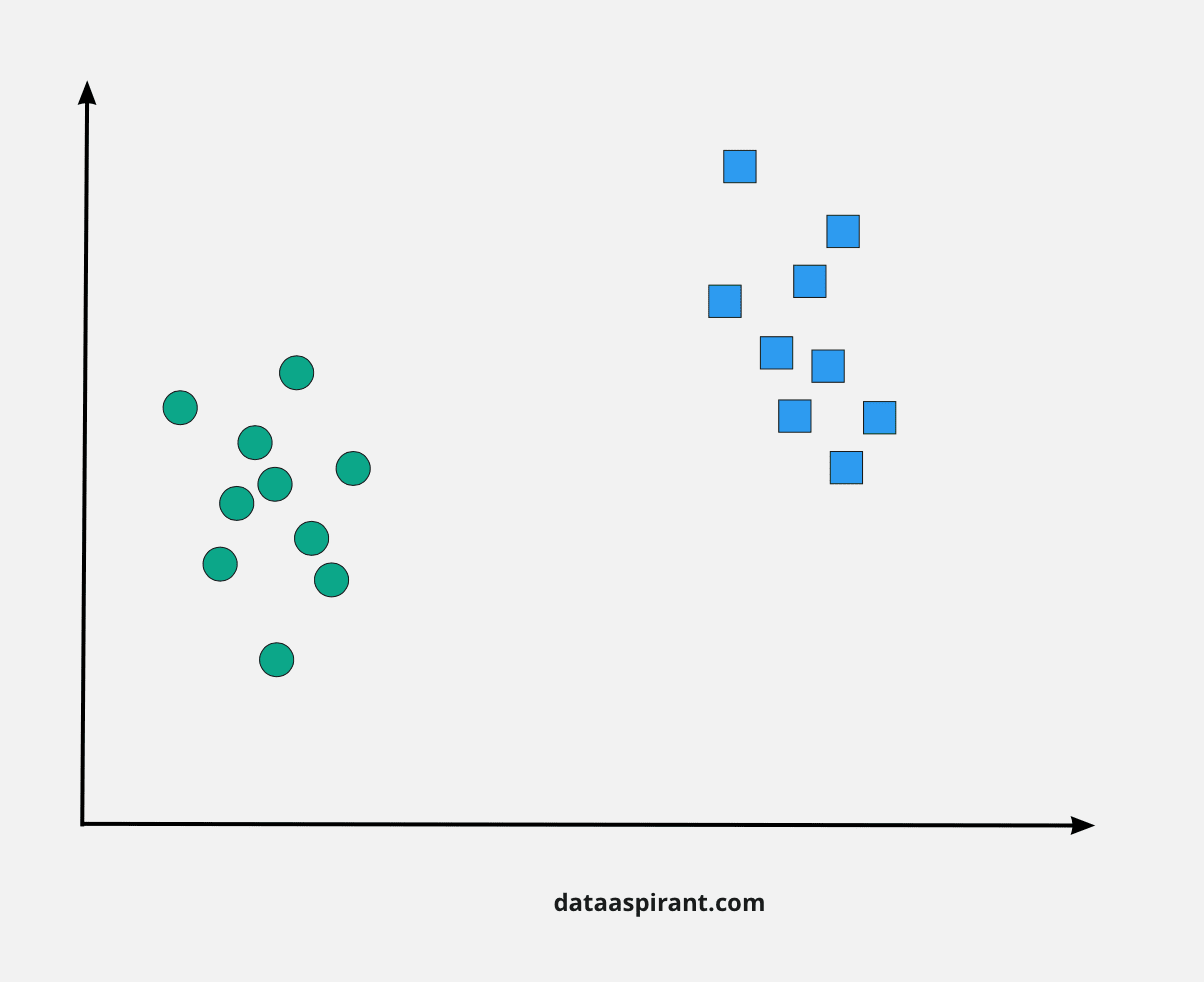Not rocket science, right?

But, as you notice, there isn’t a particular line that does this work. In fact, we have multiple lines that can separate these two classes.

So,

How does SVM find the best line to segregate the classes???

Let’s take some probable candidates and sort the things.We have three lines here. Which line as per you best separates the data?

If you’re selecting the middle line, then fantastic because that’s the line we are searching for. It can be observed more easily in this case than in the other two lines.

But, we’d like something concrete to repair our line. Though they can classify the datasets, too, it’s not a generalized line, and in machine learning, our main target is to look for a more generalized separator.

### How does the SVM find the best line?

According to the SVM algorithm, we search for the points nearest the decision boundary from both the classes. These points are called support vectors.

Now, we have to calculate the distance between the line and the support vectors. This distance is known as the margin. Our target is to maximize the margin

Svm always looks for optimal margin, i.e., for which the margin is maximum.

Now, you must be wondering why svm tries to keep the maximum separation gap between the two classes. It is done so that the model can efficiently predict future data points.

Well, it was a bit simple to segregate the above dataset. What if our dataset is a bit complex.

Let me explain with an example.So, now you can see that this dataset is not linearly separable. Just by drawing a line, you cannot classify this dataset. When solving real-world problems, you will get such types of non-linear data.

It’s clear that we cannot classify the dataset by a linear decision boundary, but, this data can be converted into a linear one using higher dimensions

Surprising, right?

Let’s create one more dimension and name it as z.

Hold on!,

How to calculate dimensions for z?

Well, it can be done by using the following equation

Z = x^2+y^2    – equation(1)

By adding this dimension, we will get three-dimensional space.

Let’s see how it will looks like.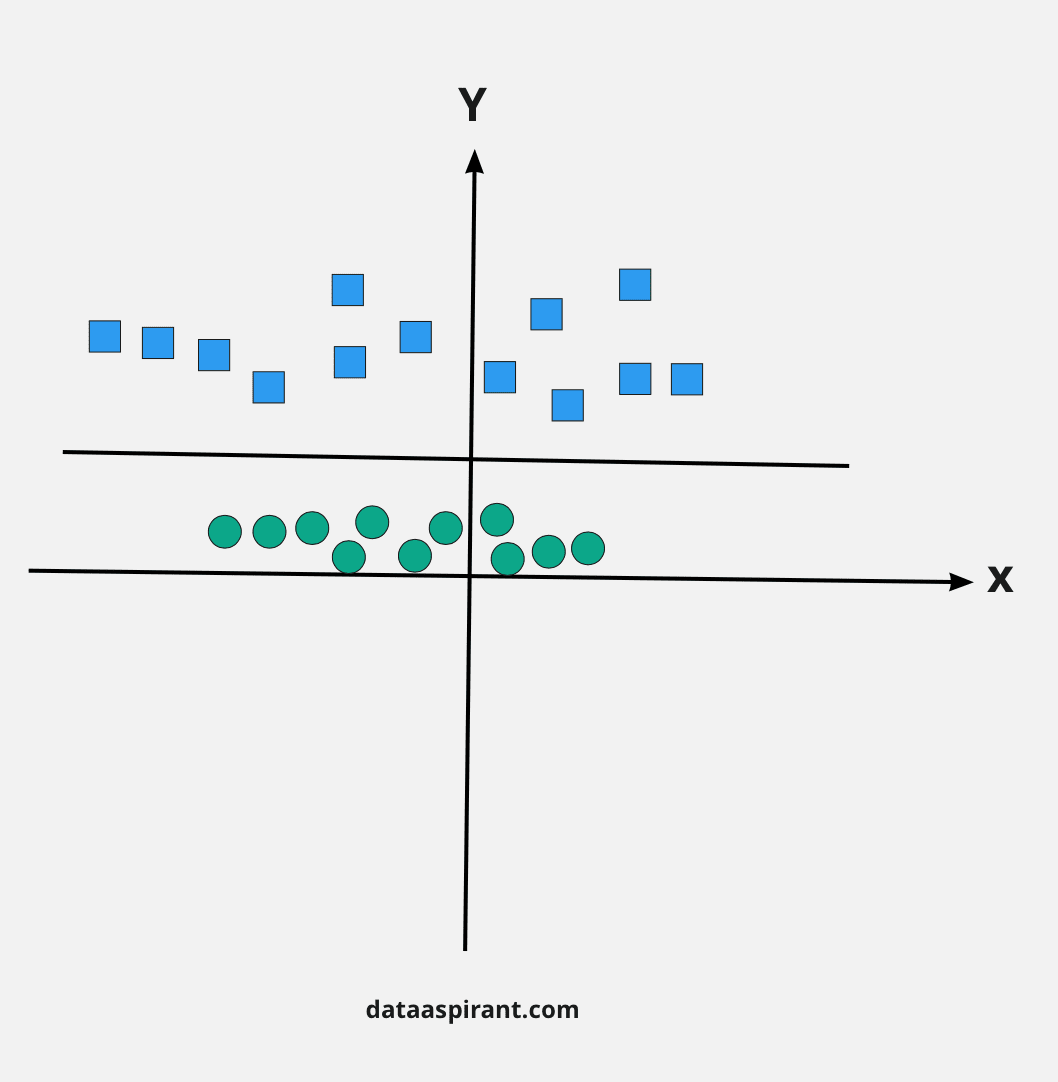Now you can see that the data has become linearly separable. As we are in three-dimensions now, the hyperplane we got is parallel to the x-axis at a particular value of z(say d).

So we have, d = x^2+y^2   (from equation 1)

We can see that it is the equation of a circle. Hence, we can convert our linear separator in higher dimensions back to the original dimensions using this equation.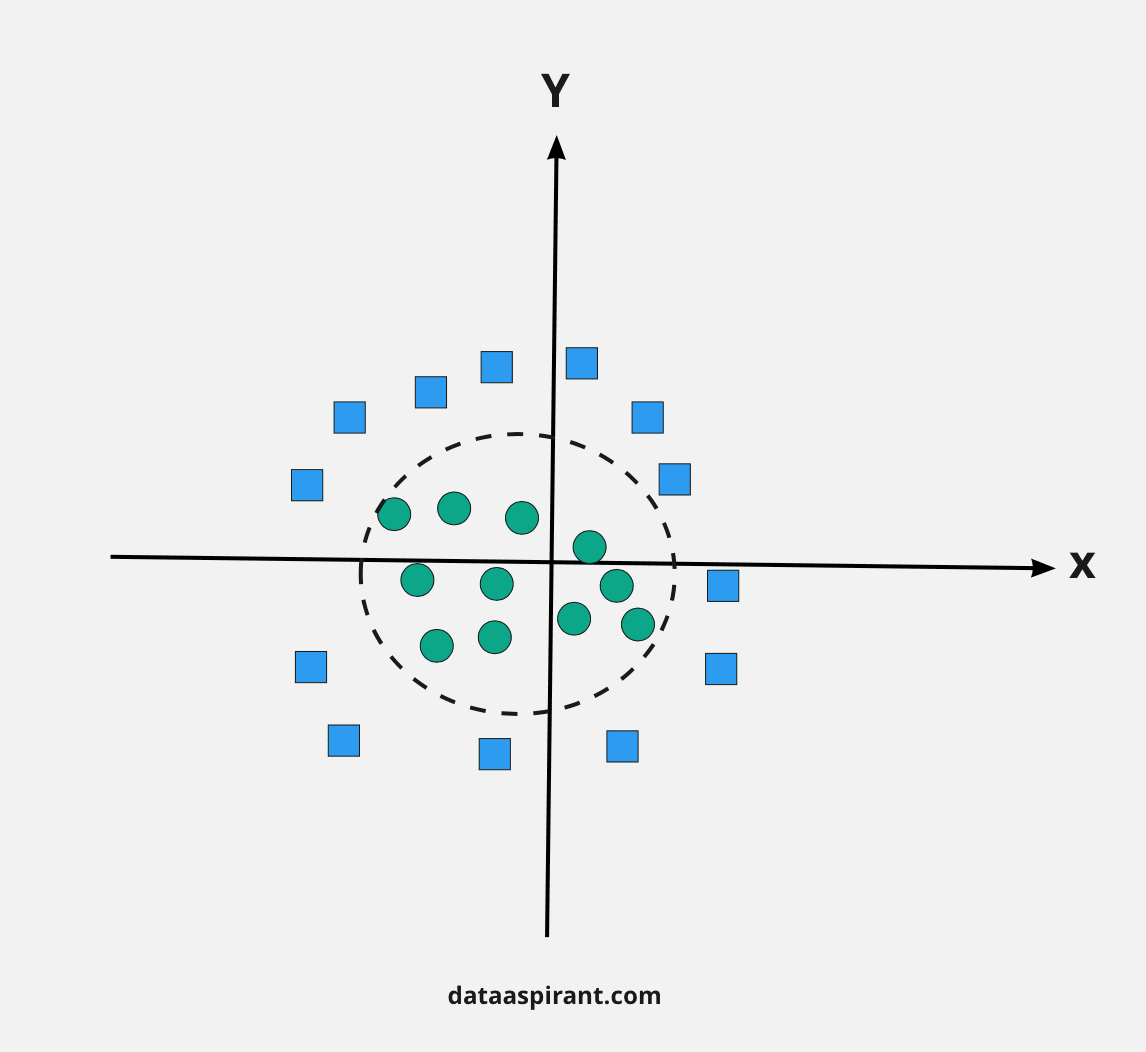Yayy, here we go. Our decision boundary or hyperplane is a circle, which separates both the classes efficiently.

In the SVM classifier, it’s easy to make a linear hyperplane between these two classes. But, another curious question which arises is,

Do we have to implement this feature by own to make a hyperplane?

The SVM algorithm takes care of that by using a technique called the kernel trick. The SVM kernel could be a function that takes low dimensional input space and transforms it into a better dimensional space, i.e., it converts non-separable problems to separable problems.

It helps us to deal with non-linear separation problems. Simply put, it does some extremely complex data transformations, then finds out the method to separate the data points based on the target classes you’ve defined.

I guess now everything is sorted regarding svm logic. Let’s see why and where we use SVMs.

## SVM Applications

SVMs are utilized in applications like

That’s why we prefer SVMs in various machine learning applications. Also, it can handle both classification and regression on linear and non-linear data.

Another reason we use SVMs is because they help us to find complex relationships among the provided dataset without you involving in plenty of transformations on your own.

It is a great algorithm to choose when you are working with smaller datasets that have tens to many thousands of features. They typically find more accurate results when put next to other algorithms due to their ability to handle small, complex datasets.

Finally, we are clear with various aspects of svm. Now, let’s dive deep and read about the most useful feature of the svm algorithm.

What’s that ??

It’s none other than kernels. Kernels help a lot when we have to deal with complex datasets. Their job is to get data as input and transform it into any required form.

They’re significant in SVM as they assist in determining various important things.

## SVM Kernel Functions

SVM algorithms use a group of mathematical functions that are known as kernels. The function of a kernel is to require data as input and transform it into the desired form.

Different SVM algorithms use differing kinds of kernel functions. These functions are of different kinds—for instance, linear, nonlinear, polynomial, radial basis function (RBF), and sigmoid.

The most preferred kind of kernel function is RBF. Because it’s localized and has a finite response along the complete x-axis.

The kernel functions return the scalar product between two points in an exceedingly suitable feature space. Thus by defining a notion of resemblance, with a little computing cost even in the case of very high-dimensional spaces.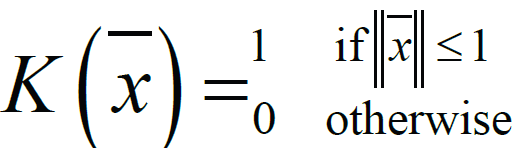## Popular SVM Kernel Functions

### Linear Kernel

It is the most basic type of kernel, usually one dimensional in nature. It proves to be the best function when there are lots of features. The linear kernel is mostly preferred for text-classification problems as most of these kinds of classification problems can be linearly separated.

Linear kernel functions are faster than other functions.

#### Linear Kernel Formula

F(x, xj) = sum( x.xj)

Here, x, xj represents the data you’re trying to classify.

### Polynomial Kernel

It is a more generalized representation of the linear kernel. It is not as preferred as other kernel functions as it is less efficient and accurate.

#### Polynomial Kernel Formula

F(x, xj) = (x.xj+1)^d

Here ‘.’ shows the dot product of both the values, and d denotes the degree.

F(x, xj) representing the decision boundary to separate the given classes.

### Gaussian Radial Basis Function (RBF)

It is one of the most preferred and used kernel functions in svm. It is usually chosen for non-linear data. It helps to make proper separation when there is no prior knowledge of data.

F(x, xj) = exp(-gamma * ||x – xj||^2)

The value of gamma varies from 0 to 1. You have to manually provide the value of gamma in the code. The most preferred value for gamma is 0.1.

### Sigmoid Kernel

It is mostly preferred for neural networks. This kernel function is similar to a two-layer perceptron model of the neural network, which works as an activation function for neurons.

It can be shown as,

#### Sigmoid Kenel Function

F(x, xj) = tanh(αxay + c)

### Gaussian Kernel

It is a commonly used kernel. It is used when there is no prior knowledge of a given dataset.

#### Gaussian Kernel Formula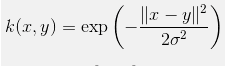### Bessel function kernel

It is mainly used for removing the cross term in mathematical functions.

#### Bassel Kernel Formula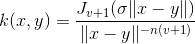Here J is the Bessel function.

### ANOVA kernel

It is also known as a radial basis function kernel. It usually performs well in multidimensional regression problems.

#### Anova Kernel Formula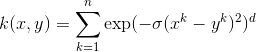## Implementing SVM Kernel Functions In Python

We have discussed the theoretical information about the kernel functions so far. Let’s see the  practical implementation to get the proper grip on the concept.

Here, we will be using the scikitlearn iris dataset.

The first step is importing the required packages

Then we splitting the loaded data into features and target data. Following the we written code to plot that.

Now Let’s implement few of the svm kernel functions we discussed in this article.

### Linear Kernel Implementation

Now we will make our svc classifier using a linear kernel.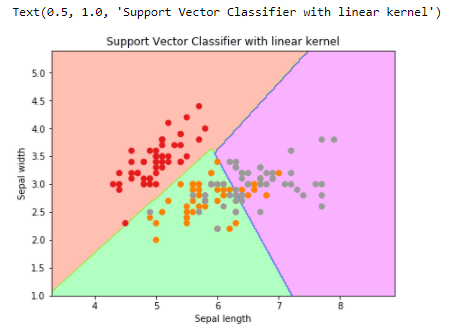### Sigmoid Kernel Implementation

Now we will make our svc classifier using the sigmoid kernel.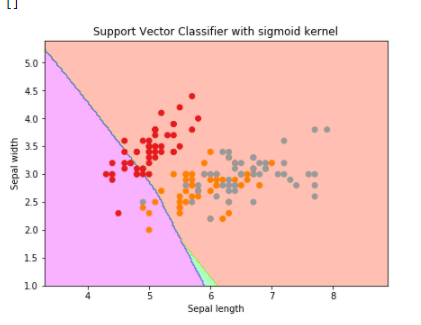### RBF Kernel Implementation

Now we will make our svc classifier using rbf kernel.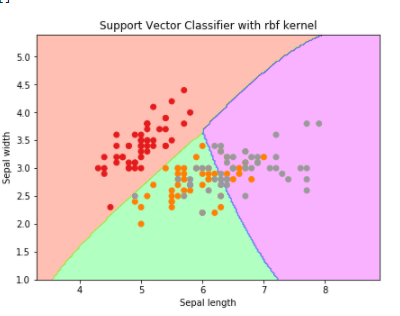### Polynomial Kernel Implementation

Now we will make our svc classifier using a polynomial kernel.There are various kernels you can use for your project. It totally depends on you and the problem you’re solving.

Like if you have to meet certain constraints, speed up the training time, or you have to tune the parameters

## How to choose the best SVM kernel for your dataset

I am well aware of the fact that you must be having this question how to decide which kernel function will work efficiently for your dataset.

It totally depends on what problem you’re actually solving. If your data is linearly separable, without a second thought, go for a linear kernel.

Because a linear kernel takes less training time when compared to other kernel functions.

• The linear kernel is mostly preferred for text classification problems as it performs well for large datasets.
• Gaussian kernels tend to give good results when there is no additional information regarding data that is not available.
• Rbf kernel is also a kind of Gaussian kernel which projects the high dimensional data and then searches a linear separation for it.
• Polynomial kernels give good results for problems where all the training data is normalized.

I hope that now the svm kernel functions got pretty straightforward for you. Let’s see the advantages and disadvantages of using an svm algorithm.

• It works well on a dataset having many features.
• It provides a clear margin of separation.
• It is very effective for the dataset where the number of features are greater than the data points.
• You can specify different kernel functions to make a proper decision boundary.

• It requires very high training time, hence not recommended for large datasets.
• It is very sensitive to outliers.

## Conclusion

So, this is the end of this article. We discussed the SVM algorithm—how it works and its applications. We also learned the important concept of SVM Kernel functions in detail.

In the end, we implemented these SVM kernel functions in python. I hope you have enjoyed and gathered lots of learnings from this article.

## What next

I would suggest doing some hands-on using Python after reading this article. Do explore remaining classification algorithms on our platform that will be very useful for you.

Sharing you the other classification algorithms articles for you reference.

#### Recommended Machine Learning Courses#### Machine Learning A to Z Course#### Complete Supervised Learning Algorithms